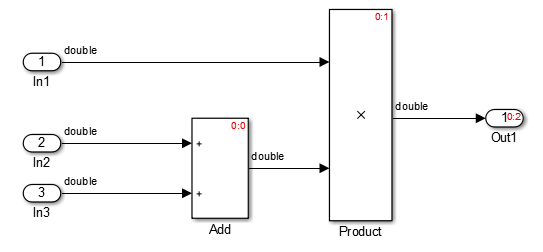Main Content

## Optimize Code by Reordering Commutable Operands

This example shows how to reorder commutable operands to make expressions left-recursive. Left -recursive reordering saves stack space in memory. This optimization improves code efficiency.

### Example Model

To reorder commutable operands, create this model and name it `operand_order`. The output signal is the result of multiplying the signal from Inport block `In1` by the sum of the signals from Inport blocks `In2` and `In3`.### Generate Code

1. Open the Model Configuration Parameters dialog box. On the Code Style tab, select the Preserve operand order in expression parameter.

2. Generate code for the model.

In the `operand_order.c` file, the `operand_order_step` function contains this code:

```operand_order_Y.Out1 = operand_order_U.In1 * (operand_order_U.In2 + operand_order_U.In3); ```

The code generator preserves the specified expression order in the model, which increases the readability of the code for code traceability.

### Generate Code with Optimization

1. Open the Model Configuration Parameters dialog box. On the Code Style tab, clear the Preserve operand order in expression parameter.

2. Generate code for the model.

In the `operand_order.c` file, the `operand_order_step` function contains this code:

```operand_order_Y.Out1 = (operand_order_U.In2 + operand_order_U.In3) * operand_order_U.In1; ```

The code generator optimizes the code by reordering the commutable operands to make the expression left-recursive, which improve code efficiency.

Download guide